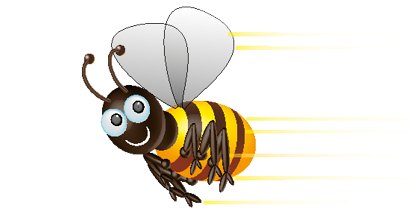Hint4 + 4 =
7 + 7 =
6 + 6 =
I must add: 6 + 5 =
Write the double:
+ + 1
+ 1